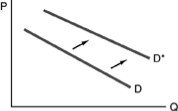• FAQ
• Contact/ Homework Answers / Economics / 71) Suppose it discovered that consumption of butter leads to
Not my Question
Flag Content

# Question : 71) Suppose it discovered that consumption of butter leads to

71) Suppose it is discovered that consumption of butter leads to a longer life. This information would lead to

A) an increase in quantity demanded.

B) an increase in demand.

C) a decrease in quantity demanded.

D) a decrease in demand.

72) Which of the following causes a decrease in demand for a normal good?

A) increase in price of a substitute

B) increase in price of a complement

C) increase in price

D) increase in income

73) Which of the following causes an increase in demand for a normal good?

A) increase in the price of a substitute

B) increase in the price of a complement

C) decrease in price

D) decrease in income74) Refer to the above figure. The rightward shift of the curve indicates

A) an increase in demand.

B) a decrease in demand.

C) an increase in quantity demanded.

D) a decrease in quantity demanded.

75) Refer to the above figure. For a normal good, the rightward shift of the curve could have been caused by

A) a technological improvement.

B) an increase in the cost of inputs.

C) an increase in income.

D) a decrease in income.

76) Refer to the above figure. The arrows show the direction of a shift in the demand curve of Good A. Which of the following would be the most likely reason for the shift in the demand curve if Good A is an inferior good?

A) an increase in the price of Good A

B) a decrease in the price of Good A

C) a decrease in consumers' income

D) an increase in consumers' income

77) Refer to the above figure for a particular good. The rightward shift of the curve could have been caused by

A) a decrease in the price of an input.

B) a decrease in the price of that good.

C) an increase in the price of a complementary good.

D) an increase in the price of a substitute good.

78) A shift of the demand curve to the left represents

A) an increase in demand.

B) a decrease in demand.

C) an increase in quantity demanded.

D) a decrease in quantity demanded.

79) Which of the following will NOT lead to a decrease in demand for a normal good?

A) an increase in income

B) an increase in the price of an input

C) a decrease in the price of a complement good

D) an increase in the number of consumers

80) A shift in the demand curve to the right represents

A) an increase in demand.

B) a decrease in demand.

C) an increase in quantity demanded.

D) a decrease in quantity demanded.

## Solution 5 (1 Ratings )

Solved
Economics 3 Months Ago 8 Views